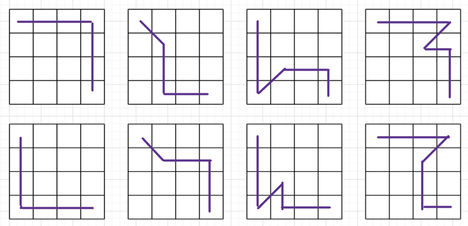# Greedy Grid GameFellow_juniorNational Girls' Programmi...

Observe the moving condition of Aliban from the statement. There are limited movements available. All possible scenarios of movements-

1. For $n=1$ or $m=1$ only $1$ path exists to reach the destination.

2. For $n=2$ and $m=2$ there are $4$ possible paths.

3. For $n=2$ or $m=2$ there are $6$ possible paths.

4. For $n>2$ and $m>2$ there are $8$ possible paths.

Look into the below image for a better understanding —Finally, iterate over all paths to calculate the score. From all scores, the maximum score will be the answer.

Analysis and solutions: link to repository.### Statistics

33% Solution Ratio
rkb_rdEarliest, 7M ago
Musarrat_18Fastest, 0.0s
still_optimisticLightest, 5.5 MB
chrootShortest, 1295B